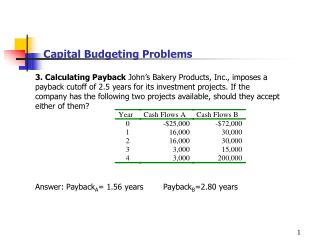DownloadDownload PresentationCapital Budgeting Problems

# Capital Budgeting Problems

Télécharger la présentation## Capital Budgeting Problems

- - - - - - - - - - - - - - - - - - - - - - - - - - - E N D - - - - - - - - - - - - - - - - - - - - - - - - - - -
##### Presentation Transcript

1. Capital Budgeting Problems • 3. Calculating Payback John’s Bakery Products, Inc., imposes a payback cutoff of 2.5 years for its investment projects. If the company has the following two projects available, should they accept either of them? • Answer: PaybackA= 1.56 years PaybackB=2.80 years

2. Capital Budgeting Problems • 4. Calculating Discounted Payback An investment project has • annual cash inflows of \$500, \$600, \$700, and \$800, and a discount • rate of 10 percent. What is the discounted payback period for • these cash flows if the initial cost is \$1,000? • Answer: Discounted PaybackA= 2.09 years

3. Capital Budgeting Problems • 12. NPV versus IRR The Heitman Group, Inc., has identified the • following two mutually exclusive projects: • a. What is the IRR for each of these projects? If you apply the IRR decision • rule, which project should the company accept? Is this decision necessarily • correct? • b. If the required return is 9 percent, what is the NPV for each of these • projects? Which project will you choose if you apply the NPV decision rule? • c. Over what range of discount rates would you choose Project L? Project S? • At what discount rate would you be indifferent between these two projects? • Answer: IRRL= 10.12% IRRS=11.46% NPVL=\$336.67 NPVS=\$377.54 • Crossover rate=8.73%

4. Capital Budgeting Problems • 13. NPV versus IRR Consider the two mutually exclusive • projects below: • Sketch the NPV profiles for M and N over a range of discount rates • from zero to 25 percent. What is the crossover rate for these two • projects? • Answer: Crossover rate=11.24%

5. Capital Budgeting Problems • 29. NPV Intuition Projects A and B have the same cost, and • both have conventional cash flows. The total undiscounted cash • inflows for A are \$1,900, and for B the total is \$1,500. The IRR for • A is 18 percent; the IRR for B is 15 percent. What can you • deduce about the NPVs for Projects A and B? What do you know • about the crossover rate? Under what circumstances would you • choose Project A over Project B? How about B over A?

6. Capital Budgeting Problems • 33. NPV Valuation The Grim Reaper Corporation wants to set up • a private cemetery business. The cemetery project will provide a • net cash inflow of \$25,000 for the firm during the first year, and • these cash flows are projected to grow at a rate of 6 percent per • year forever (the Grim Reaper keeps pretty busy). The project • requires an initial investment of \$400,000. • a. If the Grim Reaper requires a 12 percent return on investment, should the cemetery business be started? • b. The company is somewhat unsure about the assumption of a 6 percent growth rate in its cash flows. At what constant growth rate would the company just break even if it still requires a 12 percent return on investment? • Answer: NPV=\$16,667 g=5.75%